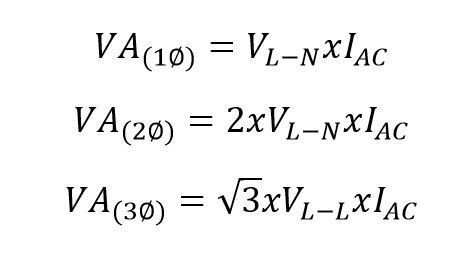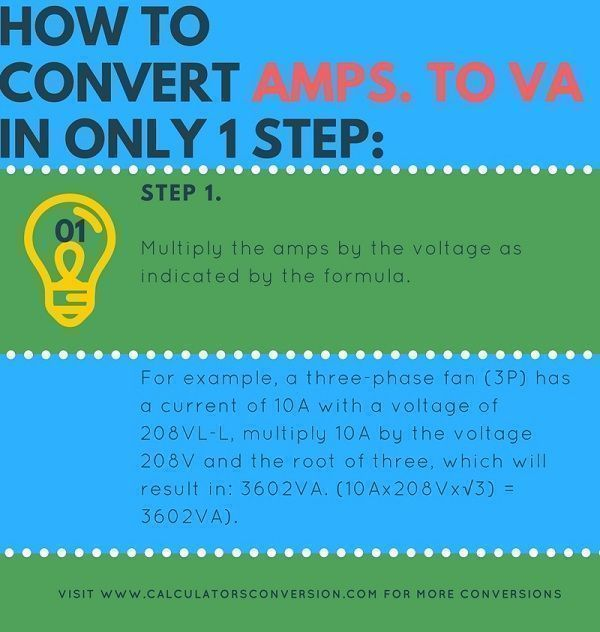# Amperes to VA – Convert, calculator, chart and formula

With this calculator you can convert from amperes to VA automatically, easily, quickly and free any electric power.

We also show how to convert Amp. to VA to 1 step, the formula that is used for the conversion and a chart with the main conversions from Amp. to VA.

## Amp. to VA calculation formula for generator, UPS and transformer:• IAC=Ampere.
• VL-L=Volts Line-Line.
• VL-N=Volts Line-Neutral.
• VA=Volt-Ampere.

## How to convert Amp. to VA in only 1 step:Step 1:

Multiply the amps by the voltage indicated in the formula. For example, a three-phase fan (3P) has a current of 10A with a voltage of 208VL-L, then multiply 10A by the voltage 208V and the root of three, which will result in: 3602VA. (10Ax208Vx√3) = 3602VA).

## Definition Amperes and S (VA):

VA: A Volt-ampere, commonly referred to as a VA, is commonly used as a unit of power in obtaining the electrical capacity of circuit breakers, uninterrupted power supplies and wirings.

VA is larger than Watts because loads are inductive such as motors, discharge lighting, reactors and more current is required to keep the magnetic field energized than is -turned into heat (W).

Inductive devices or loads such,. as tansformers and motors having power factor less than 1.0 are generally rated in VA.

Ampere: Amperage is a term often used by electricians, and means electrical current, measured in amperes, or amps. The ampere is the SI unit for electrical current, or the amount of electrical charge that flows through a conductor in a given time. One ampere is a charge of one coulomb — about 6.241 X 1018 electrons — per second flowing past a given point.

## Amps to VA conversion chart:

 Amp. VA Fases Volt 1Amp. 360,26VA 3F 208Volt 2Amp. 720,53VA 3F 208Volt 3Amp. 1080,79VA 3F 208Volt 4Amp. 1441,06VA 3F 208Volt 5Amp. 1801,33VA 3F 208Volt 6Amp. 2161,59VA 3F 208Volt 7Amp. 2521,8VA 3F 208Volt 8Amp. 2882,132VA 3F 208Volt 9Amp. 3242,39VA 3F 208Volt 10Amp. 3810,51VA 3F 220Volt 20Amp. 7621,02VA 3F 220Volt 30Amp. 11431,53VA 3F 220Volt 40Amp. 15242,04VA 3F 220Volt 50Amp. 19052,55VA 3F 220Volt 60Amp. 22863,07VA 3F 220Volt 70Amp. 26673,5VA 3F 220Volt 80Amp. 30484,09VA 3F 220Volt 90Amp. 34294,6VA 3F 220Volt 100Amp. 76210,23VA 3F 440Volt 200Amp. 152420,4VA 3F 440Volt 300Amp. 228630,7VA 3F 440Volt 400Amp. 304840,9VA 3F 440Volt 500Amp. 381051,17VA 3F 440Volt 600Amp. 457261,4VA 3F 440Volt 700Amp. 533471,6VA 3F 440Volt 800Amp. 609681,88VA 3F 440Volt 900Amp. 685892,11VA 3F 440Volt 1000Amp. 796743,37VA 3F 460Volt 2000Amp. 1593486,74VA 3F 460Volt 3000Amp. 2390230,114VA 3F 460Volt 4000Amp. 3186973,48VA 3F 460Volt 5000Amp. 3983716,85VA 3F 460Volt

Spanish version Amperios a VA
Rate this calculator Amp. to VA:  [kkstarratings]